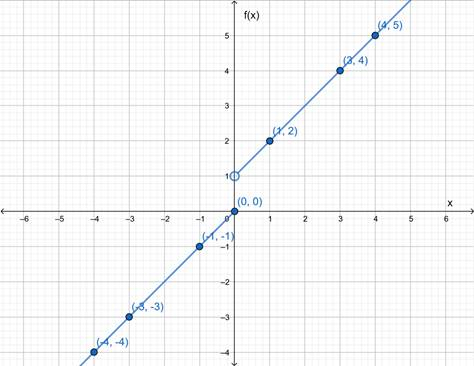# The graph of the given function.### Precalculus: Mathematics for Calcu...

6th Edition
Stewart + 5 others
Publisher: Cengage Learning
ISBN: 9780840068071### Precalculus: Mathematics for Calcu...

6th Edition
Stewart + 5 others
Publisher: Cengage Learning
ISBN: 9780840068071

#### Solutions

Chapter 2.2, Problem 37E
To determine

## To sketch : The graph of the given function.

Expert Solution

### Explanation of Solution

Given information :

f(x)={x        if  x0x+1    if  x>0

Graph :

 x 0 1 −1 −2 −3 −4 −6 f(x) 5 5 5 5 4 5 7The above curve is the graph of the given piecewise defined equation.

Interpretation : The part of the graph to the left of x=0 coincides with the graph of f(x)=x and the part of the graph to the right of x=0 coincides with the graph of f(x)=x+1 .

### Have a homework question?

Subscribe to bartleby learn! Ask subject matter experts 30 homework questions each month. Plus, you’ll have access to millions of step-by-step textbook answers!